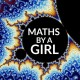# 3: Dirichlet’s Unit Theorem

Last time, I discussed Dedekind’s Criterion. In this post I aim to introduce Dirichlet’s Unit Theorem.

Let L be a number field (finite extension of the field of rational numbers).

We will define a complex embedding of L as being a field homomorphism:Then a real embedding of L  is similarly defined as being a field homomorphism:We note that the complex embeddings can be given in pairs:where we obtain one embedding by taking the complex conjugate of the other embedding in the pair.

Hence, given a number field L, we can label its real embeddings asand its complex embeddings asNoting that the degree, n, of the field extension L/Q (where Q is the field of rational numbers) is equal to the total number of embeddings and hence we deduce that n = 2s + r. The fact that there is this equivalence is non-trivial, so don’t worry if you don’t immediately see why this is! In order to prove this we must use tools from Galois Theory, such as something called the ‘Tower Law’, and therefore I will emit the proof from this blog post.

Let me know if you’re interested in seeing Galois Theory related posts in the future!

Lettingbe the units in the ring of algebraic integers of L we are now ready to state Dirichlet’s Unit Theorem.

## Dirichlet’s Unit TheoremThe proof of this is rather complicated and involves defining a map, whose kernel is this finite cyclic group, which takesinto a lattice. Considering lattices are involved in the proof, you can start to see where the isomorphism with the integers comes from.

## Why is this useful?

Take the case where r = 0 and s = 1, i.e. L is an imaginary quadratic number field:As r + s – 1 = 0, we get thatis a finite group. By explicitly defining the isomorphism we can deduce that the finite cyclic group is simply {+/- 1}, andis generated by one element – the fundamental unit, i.e.I’ve purposefully skipped a lot of the details as what is important is the result. The proof requires a bit of magic and is not terribly enlightening. However, the result is extremely important – using this, for the case of imaginary quadratic fields, we can completely specify, provided we find the fundamental unit.

## Finding the Fundamental Unit

It turns out that finding the fundamental unit in this case is extremely straightforwards. There are various different cases.

• If d = 2, 3 (mod 4) we characterise the fundamental unit u in the following way: let b be the least positive integer such that db2 + 1 or db2 – 1 is of the form a2 for some natural number a. Then:• If d = 1 (mod 4) and d is not equal to 5: let b be the least positive integer such that db2 + 4 or db2 – 4 is of the form a2 for some natural number a. Then:• If d = 5:(If you would like the proof as to WHY this is true, leave me a comment below and I’ll be happy to make a post!)

#### Example: d = 2

Then 𝑏 = 1 works since 2 − 1 = 1so 1 + √2 is a fundamental unit.

In order to get a lot of the symbols I had to LaTex some of the sections so sorry if the formatting is a little off! Next time we will start solving some equations using algebra!

M x

## 3 thoughts on “3: Dirichlet’s Unit Theorem”

1.Ingwald says:

Did you really screenshoot equations all this time to add them to post? You’re truly the most patient human being I ever heard of. Formatting is fine, so don’t worry.
So ashamed that I didn’t go that far with Warden’s Algebra while you’re heading to cover chapter 8 contents. 😦 Posts on Galois Theory are most welcome, and I have a strong feeling that bits of Galois Theory can’t be avoided in you future blog posts.
Post on detailed proof is also welcome in case you have some insights/shortcuts/useful results to share, personally I don’t want you to retype books for ones who are to lazy to google.
I’m glad that you post more often. Keep it up! And thank you so much for your efforts.

Liked by 1 person

1.mathsbyagirl says:

Thank you for your nice comment! I’m trying to not assume too much algebraic knowledge but if anything is unclear I’m happy to (try) and explain. I am however keen on exploring Galois Theory, it ties in perfectly with Algebraic Number Theory!

Like